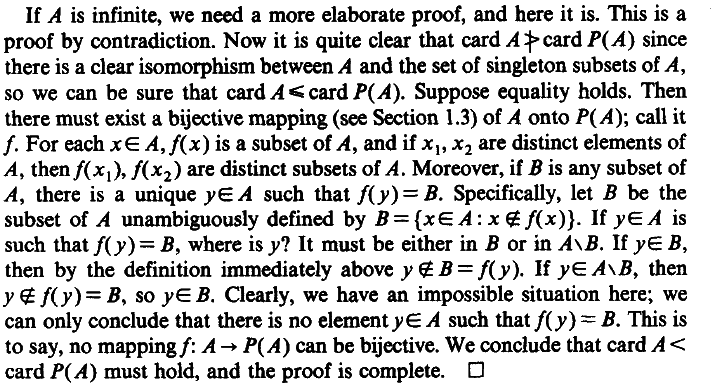I was studying the first chapter "Sets and Structures" of the "A Course in Advanced Calculus - Robert S. Borden". I faced a difficulty at the part of the proof of contradiction.I got confused at what this $B= \{x \in A : x \not\in f(x) \}$ is and
how it's true that $If~y \in A ~\text{is such that}~f(y)=B, \text{where is y? It must be either in}~B~\text{or in} A \setminus B.$
Can anyone explain what's going on here?

Assuming that ##B## is in the range of ##f##, there is ##y\in A## such that ##f(y)=B##. Forget about what ##f## and ##y## and ##B## are for a moment; that's just the definition of range from pre-calc. Then either ##y\in f(y)=B## or ##y\not\in f(y)=B## (note that ##y\not\in B\Rightarrow y\in A \setminus B##); remember, since ##f(y)=B## is a subset of ##A## and ##y\in A##, one of those has to be true. But either way you go, you end up with an absurdity; either $$y\in f(y)\Rightarrow_1 y\in B\Rightarrow_2 y\not\in f(y)$$ or $$y\not\in f(y)\Rightarrow_2 y\in B\Rightarrow_1 y\in f(y)$$ where the ##\Rightarrow_1## implications are "true" by virtue of the fact that ##f(y)=B## and the ##\Rightarrow_2## implications are true from the definition of ##B=\{x\in A:x\not\in f(x)\}##.